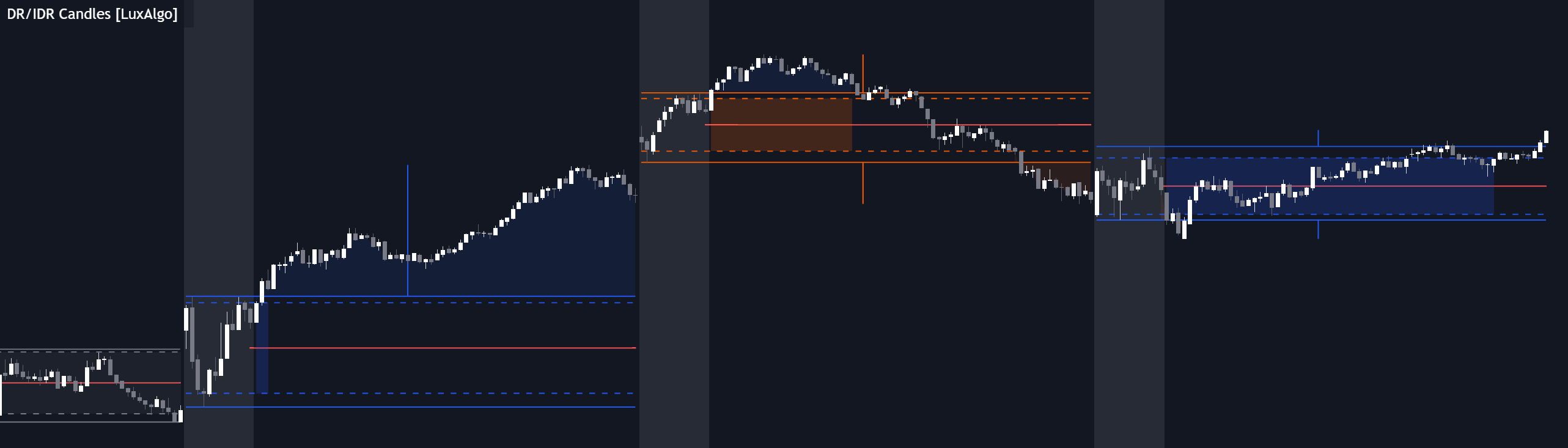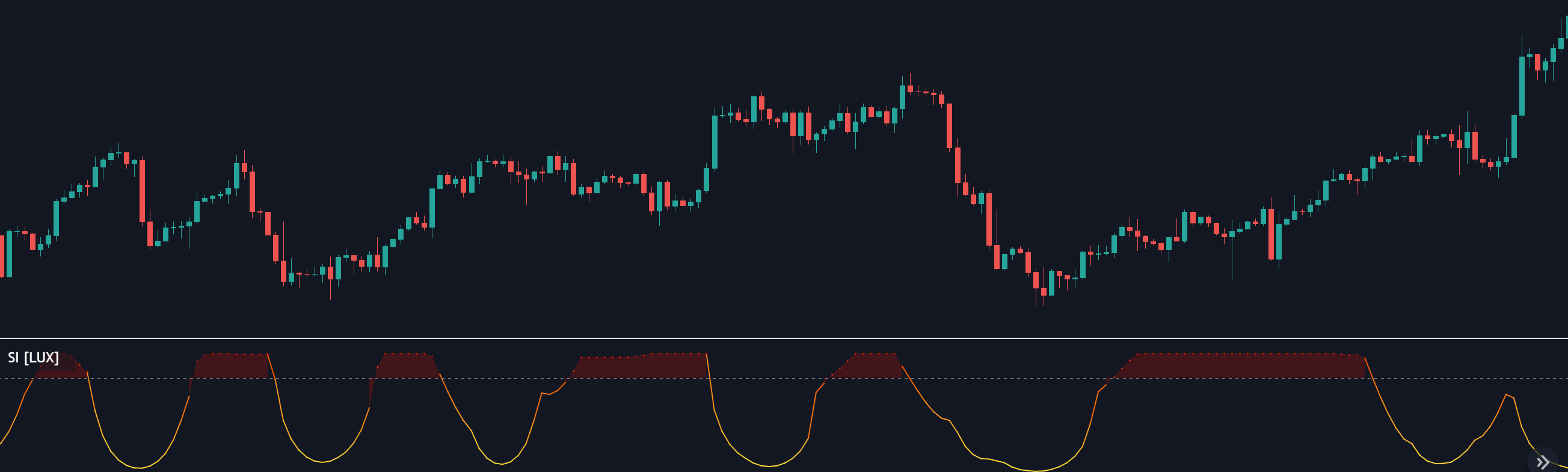# Squeeze Index

The Squeeze Index aims to measure the action of price being squeezed, and is expressed as a percentage, with higher values suggesting prices are subject to a higher degree of compression.

The Squeeze Index aims to measure the action of price being squeezed, and is expressed as a percentage, with higher values suggesting prices are subject to a higher degree of compression.

#### Settings

• Convergence Factor: Convergence factor of exponential envelopes .
• Length: Period of the indicator.
• Src: Source input of the indicator.

#### Usage

Prices being squeezed refer to the action of price being compressed within a tightening area. Prices in a tight area logically indicate a period of stationarity, price breaking out of this area will generally indicate the trader whether to buy or sell depending on the breakout direction.

The convergence factor and length settings both play an important role in the returned indicator values. A convergence factor greater than the period value will detect more squeezed prices area, while a period greater than the convergence will return fewer detected squeezed areas.

We recommend using a convergence factor equal to the period setting or a convergence factor twice as high.

The above chart makes use of a convergence factor of 100 and a period of 10.

Due to the calculation method, it is possible to see retracements being interpreted as price squeezing. This effect can be emphasized with higher convergence factor values.

#### Details

In order to measure the effect of price being squeezed in a tighter area we refer to damping, where the oscillations amplitude of a system decrease over time. If the envelopes of a damped system can be estimated, then getting the difference between the upper and lower extremity of these envelopes would return a decreasing series of values.

This approach is used here. First the difference between the exponential envelopes extremities is obtained, the logarithm of this difference if obtained due to the extremities converging exponentially toward their input.

We then use the correlation oscillator to get a scaled measurement.

## Recent posts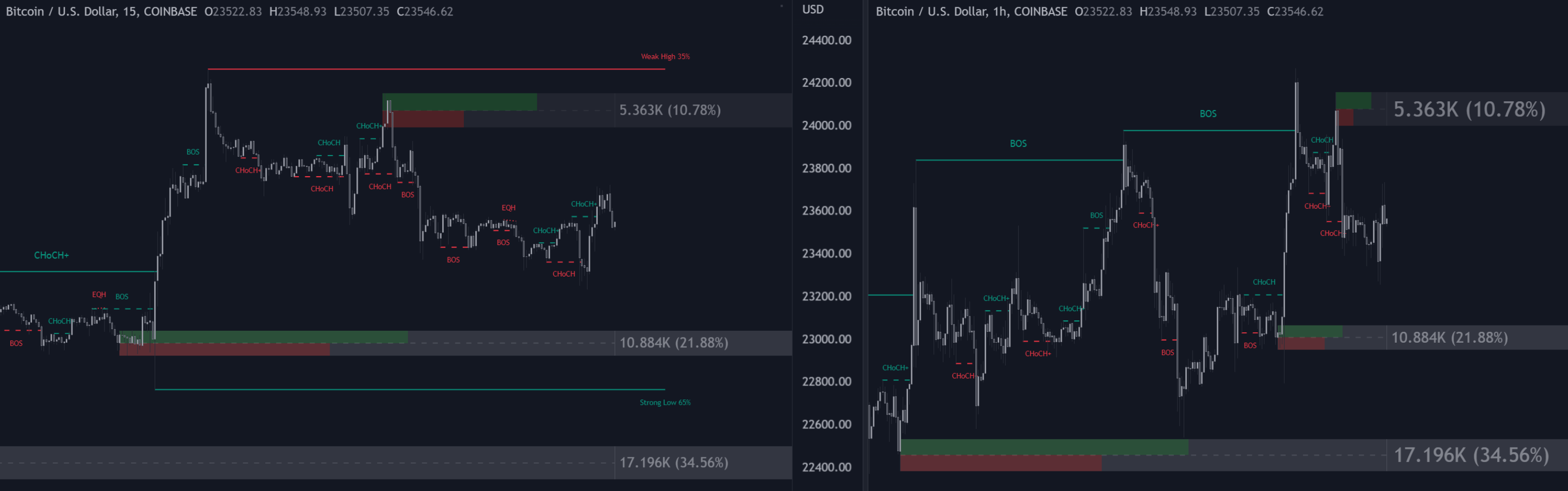### Price Action Concepts - 1.2 Update!

##### February 6, 2023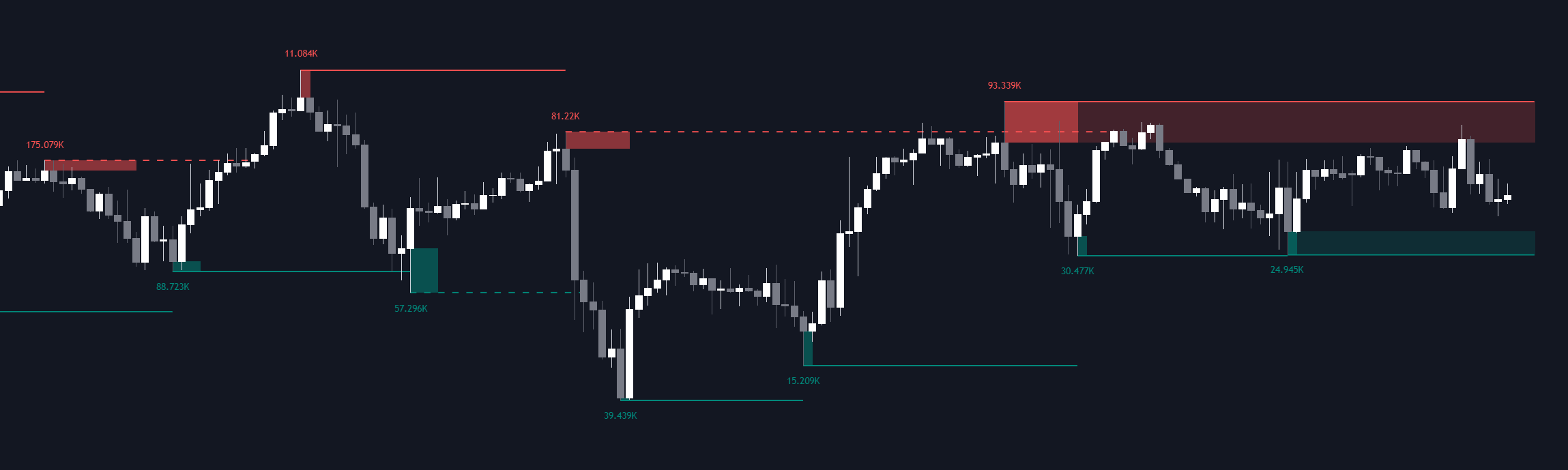### Liquidity Swings

##### January 30, 2023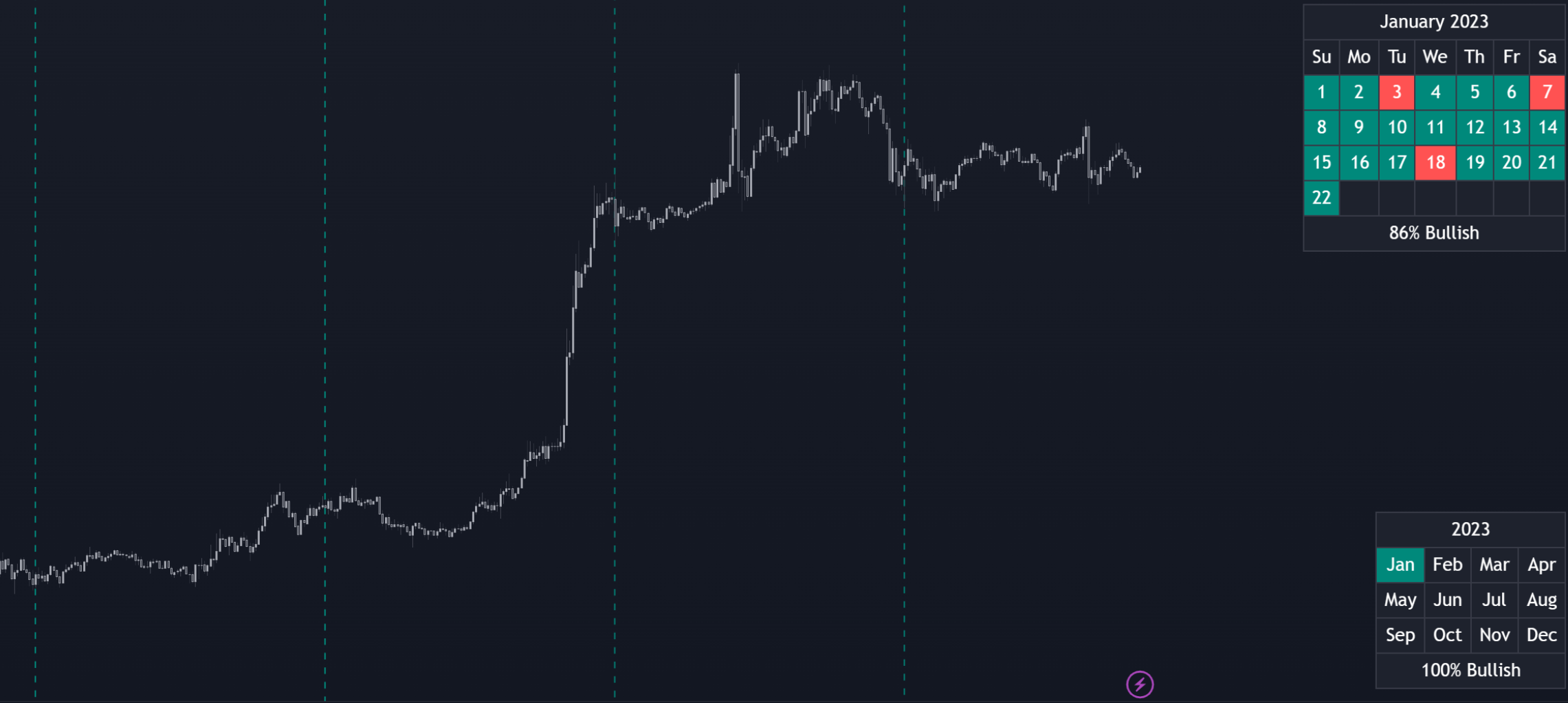##### New indicator